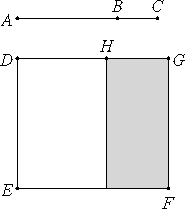# Proposition 38

If two medial straight lines commensurable in square only and containing a medial rectangle are added together, then the whole is irrational; let it be called the second bimedial straight line.

Let two medial straight lines AB and BC commensurable in square only and containing a medial rectangle be added together.

I say that AC is irrational.

I.41

Set out a rational straight line DE, and apply parallelogram DF to DE equal to the square on AC, producing DG as breadth.II.4

Since the square on AC equals the sum of the squares on AB and BC and twice the rectangle AB by BC, apply EH, equal to the sum of the squares on AB and BC, to DE. Then the remainder HF equals twice the rectangle AB by BC.

Since each of the straight lines AB and BC is medial, therefore the squares on AB and BC are also medial. But, by hypothesis, twice the rectangle AB by BC is also medial. And EH equals the sum of the squares on AB and BC, while FH equals twice the rectangle AB by BC, therefore each of the rectangles EH and HF is medial.

X.22

And they are applied to the rational straight line DE, therefore each of the straight lines DH and HG is rational and incommensurable in length with DE.

X.11

Since AB is incommensurable in length with BC, and AB is to BC as the square on AB is to the rectangle AB by BC, therefore the square on AB is incommensurable with the rectangle AB by BC.

But the sum of the squares on AB and BC is commensurable with the square on AB, and twice the rectangle AB by BC is commensurable with the rectangle AB by BC.

X.13

Therefore the sum of the squares on AB and BC is incommensurable with twice the rectangle AB by BC.

But EH equals the sum of the squares on AB and BC, and HF equals twice the rectangle AB by BC.

Therefore EH is incommensurable with HF, so that DH is also incommensurable in length with HG.

X.36

Therefore DH and HG are rational straight lines commensurable in square only, so that DG is irrational.

But DE is rational, and the rectangle contained by an irrational and a rational straight line is irrational, therefore the area DF is irrational, and the side of the square equal to it is irrational.

But AC is the side of the square equal to DF, therefore AC is irrational. Let it be called a second bimedial straight line.

Q.E.D.

## Guide

This proposition is used in X.44, X.56, X.62, and X.67.